# MalwareBytes CrackMe #2 Write-Up

###### May 19, 2018

MalwareBytes recently released their second CrackMe Challenge and I managed to solve it. This article will be my write-up for it so readers can see the techniques that were used in this CrackMe and the steps I went through to reverse engineer the application and get the flag. If you want to follow along you can download the application from the official MalwareBytes post here.

Like usual, when I start an analysis on an unknown binary I always start by using rabin2 to get basic information about it. Here is the output from the -I flag: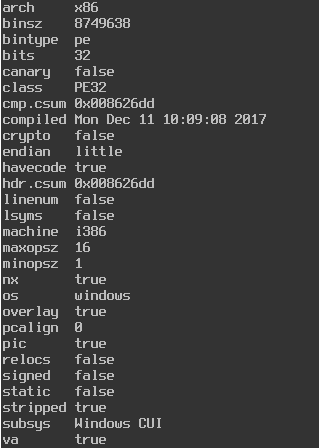We can see that this is a standard Windows executable. Now let us run the binary to see what we are looking for in this challenge.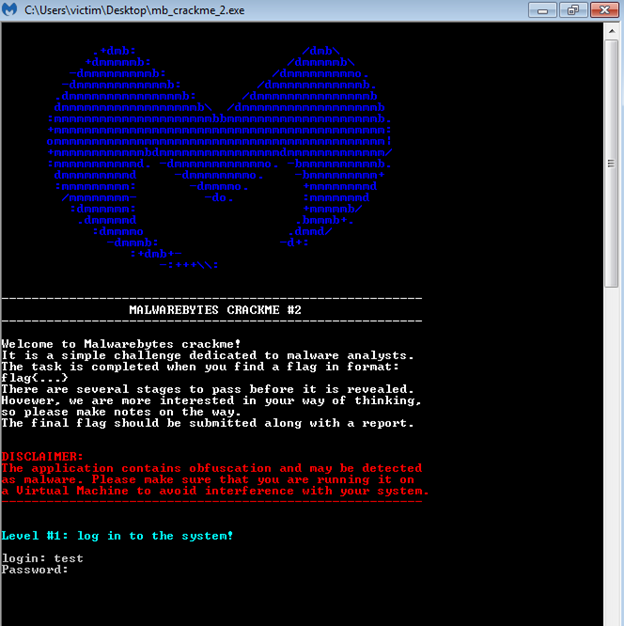As you can see, it asks for us to input a username and password. If we get it wrong, then the application closes. So, the next step would be to find that username and password.

We can use the -zqq flag to analyze the strings in the binary. Some of the more important strings I found are for Python error codes, like “Error loading Python DLL '%s'.\n” and other errors that led me to believe that this was a wrapper for a Python script. We can extract the Python code from the binary using the tool Python Exe Extractor that is freely available on GitHub.

Using this tool, I extracted all the Python libraries and files to a folder. The tool claims that the file without an extension would be the main python file of the executable, in our case that would be the file “another” (based on the file “another.exe.manifest”). This should have been a regular Python script but instead it was a compiled Python binary, so I renamed the file to “another.pyc”. I attempted to use the tool uncompyle6 to extract the python code but got the error “unknown magic number 99”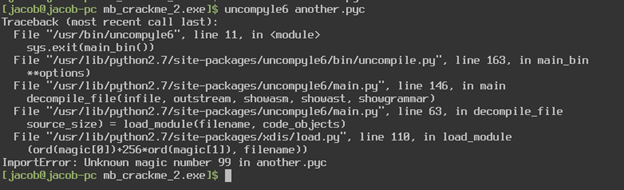The Python Exe Extractor Readme said that this problem could occur and to use the -p flag on the file to fix this issue. After running the script on the file, not only does it append the correct magic number to the file, but it also extracts the python code for me! Now we have a fully functional Python script that we can work with.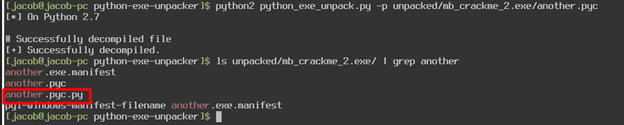Now we can start looking through the Python code and see where the username and password are stored. The main function of the script would be a good first place to start.You can see that the first thing the script does is to retrieve a key from that stage1_login function. It then verifies the key in the check_if_next function. If the key is verified, then we can move on to the next stage. Let us look at the stage1_login function and see what is going on there.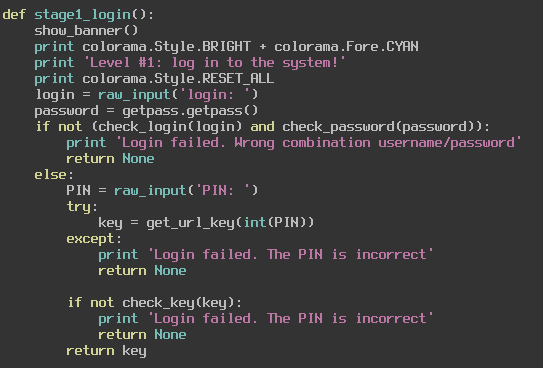We can see that it is simply grabbing the username and password from user input and sending them to their respective functions check_login and check_password. If those are verified, then it looks like we need to input a PIN. If we get that correct then we move on to the next stage of this challenge. Let us see what the check_login and check_password functions need from us before worrying about that PIN.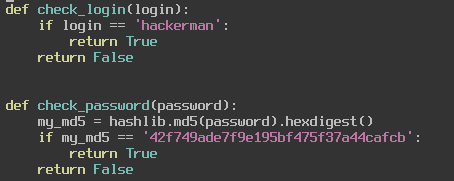The check_login function just compares our username to “hackerman” and returns True if we are logging in with that username. The check_password function is slightly more secure in the fact that it is using an md5sum to verify the password, however md5 is not the most secure hashing algorithm and we can reverse the hash and get the password “Password123”. Let us input those into the application and see what happens.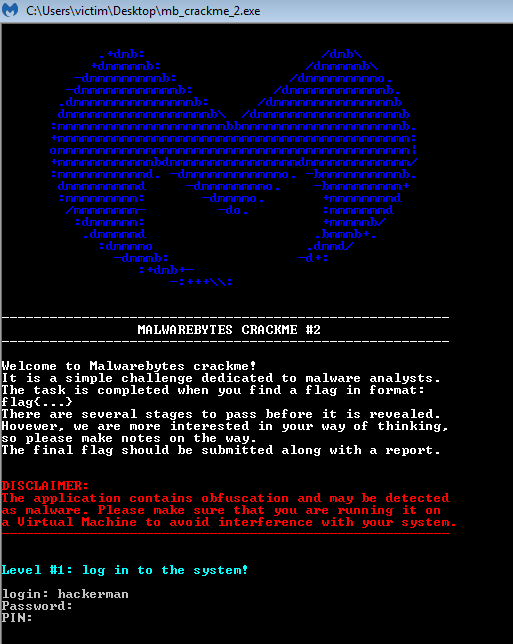By getting those correct we now move on to figuring out what this PIN is. We saw earlier that the program grabs our raw input for the PIN and passes it to the function get_url_key, then verifies the key with the function check_key. Let us look at these two functions and see what they are doing.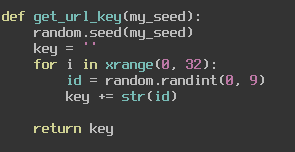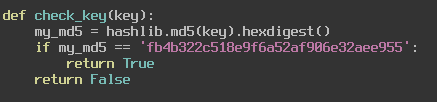You can see that the PIN we input is used as a seed for Python’s random number generator. We then generate a string of 32 random integers that are between 0 and 9 and return the key. We then pass this to our check_key function where we compare it to an md5sum that decodes to “95104475352405197696005814181948”. So, our goal here is to find a seed that will return those 32 characters. We can do this easily by bruteforcing, I created a simple python script to do this.

```import random   import hashlib i = 0 key = ''   while True: random.seed(i) key = ''   for x in range(0, 32): id = random.randint(0, 9) key += str(id) my_md5 = hashlib.md5(key).hexdigest() if my_md5 == 'fb4b322c518e9f6a52af906e32aee955':     print('Key is: ' + key)     print('PIN is: ' + str(i))     break   else:     print('PIN is not: ' + str(i))     i += 1    ```

Once ran we can see that the PIN is 9667. We can test this out on the application to see if it works.We managed to pass Level 1! Now we must figure out the second challenge which asks us to find a “secret console”. Let us go back to the Python code of the application and see exactly how it is loading up this secret console and what we must do to find it. The main function makes a call to decode_and_fetch_url, so this is where we will look first.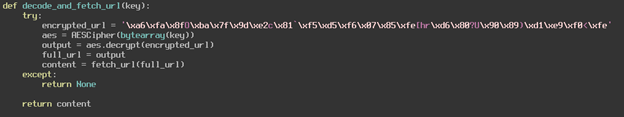This function looks like it is simply using the key “95104475352405197696005814181948” to decrypt that URL. This is easy to reverse since we know the key, I also wrote a small Python script to do just this.

```from Crypto.Cipher import AES import urllib2 import io from PIL import Image unpad = lambda s: s[:-ord(s[len(s)-1:])]```

```class AESCipher:     def __init__(self, key):         self.key = ''.join(map(chr, key))```

```    def encrypt(self, raw):         raw = pad(raw)         cipher = AES.new(self.key, AES.MODE_ECB)         return cipher.encrypt(raw)```

```    def decrypt(self, enc):         cipher = AES.new(self.key, AES.MODE_ECB)         return unpad(cipher.decrypt(enc))```

```key = '95104475352405197696005814181948' encrypted_url ='\xa6\xfa\x8fO\xba\x7f\x9d\xe2c\x81`\xf5\xd5\xf6\x07\x85\xfe[hr\xd6\x80?U\x90\x89)\xd1\xe9\xf0<\xfe' aes = AESCipher(bytearray(key)) output = aes.decrypt(encrypted_url) print('url is: ' + output)  ```

We can see that the URL is https://i.imgur.com/dTHXed7.png which is an image that looks like TV static.Continuing through the decode_and_fetch_url function we can see that there is a call to fetch_url. We will examine this function next and see exactly what it is we are fetching from the URL.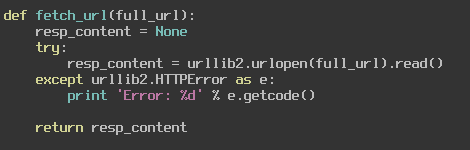This is simply grabbing the content from the webpage, in our case it is jus grabbing the picture content and returning it. In the main function we use this data to call get_encoded_data. This function uses the Python Image library to convert the weird static image into raw bytes and return it to the main function, which then passes it to load_level2 which then uses the VirtualAlloc function to create space for these raw bytes. It then moves those bytes into memory and runs it as a function. We can use a Python script to decode these raw bytes the same way the challenge does and output them to a file.

```from Crypto.Cipher import AES import urllib2 import io from PIL import Image unpad = lambda s: s[:-ord(s[len(s)-1:])]```

```class AESCipher:     def __init__(self, key):         self.key = ''.join(map(chr, key))```

```    def encrypt(self, raw):         raw = pad(raw)         cipher = AES.new(self.key, AES.MODE_ECB)         return cipher.encrypt(raw)```

```    def decrypt(self, enc):         cipher = AES.new(self.key, AES.MODE_ECB)         return unpad(cipher.decrypt(enc))```

```key = '95104475352405197696005814181948' encrypted_url ='\xa6\xfa\x8fO\xba\x7f\x9d\xe2c\x81`\xf5\xd5\xf6\x07\x85\xfe[hr\xd6\x80?U\x90\x89)\xd1\xe9\xf0<\xfe' aes = AESCipher(bytearray(key)) output = aes.decrypt(encrypted_url) print('url is: ' + output)```

`resp_content = urllib2.urlopen(output).read()`

```imo = Image.open(io.BytesIO(resp_content)) rawdata = list(imo.getdata()) tsdata = '' for x in rawdata:     for z in x:         tsdata += chr(z) del rawdata output_file = file('some_file', 'w') output_file.write(tsdata)  ```

Analyzing this file with the “file” command on Linux we can see that it is a Windows DLL file. So overall, the second stage of this challenge is getting a DLL from an image hosted online and is running that DLL to spawn some hidden console. Now we get to move on to our favorite tool, Radare2, to analyze this DLL and find where this console is. Let us run through the strings using the -zqq flag and see what looks interesting.

I found the strings “dump_the_key”, “secret_console”, and “Notepad” to be fairly interesting. One looks like a command we will need to run once we find this console, the second looks like a good reference to start at when looking for the console, and the third might be a hint as to where this console is. These are just speculations though, let us go into the application in Radare2 and see what we can find.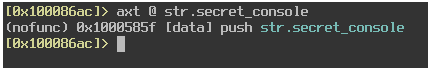After analyzing the file using the command aaaa, I find the XREF of the string “secret_console” by using the command axt @ str.secret_console. We can see that this string is pushed onto the stack at the location 0x1000585f.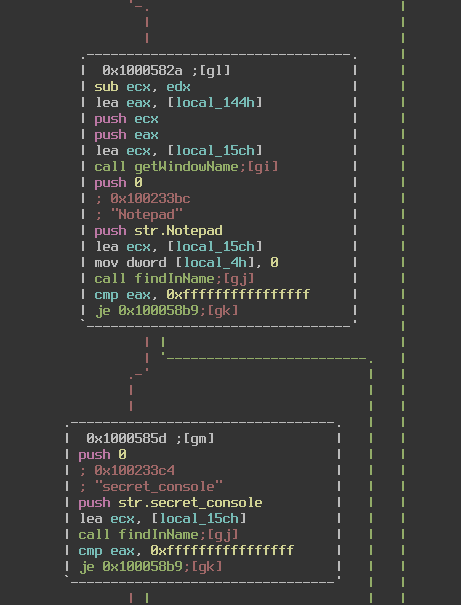At this location you can see that the “Notepad” string is also called earlier. What the code is doing basically boils down to looking through all open windows for the words “Notepad” and “secret_console” in their name. If it finds it then it waits for us to input a command, in this case “dump_the_key”. In the screenshot above I have changed the names of the functions getWindowName and findInName to better represent what they are doing.

So, all we have to do is create a text file with the name “secret_console.txt” and open it in Notepad. Then we type the command “dump_the_key” and hopefully we will pass stage2.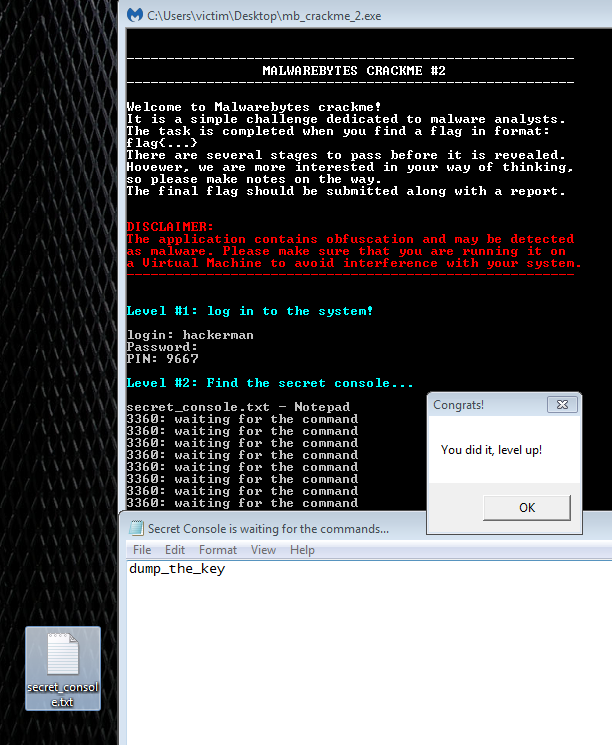Nice, now Stage 3 requires us to guess an RGB value to get the flag. This consists of three values ranging from 0 to 255. If we were to bruteforce it, that would be 16,581,375 possible combinations. Assuming we can perform 255 guesses per second that would still be around 18 hours to crack the code. I do not have that long to wait, so we can jump into the Python code and see what is in store for us there. The function we will need to analyze is the decode_pasted function.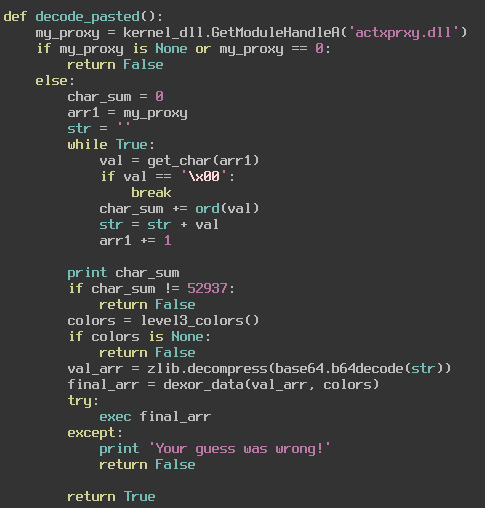This function gets the handle of actxprxy.dll and uses that handle to generate a base64 encoded, zlib compressed string. Now we could figure out this string by hand, but I decided to change the python script to print it out for me at this function. The outputted value is this:

`'eJx9lL1OAkEQx+sreIeLlUQKBPXUxAISYmehD2As/IrEGKQwMT4AJLzD5ioIhNwd9wEFhQJ+JNgo/YUg4CVEE7ji7LzdPe4GOWl+2czO/GdnZnc/jgfoamxcmvrJqHuaL2znssxgdDTUz/oWW0fLSLjRKiFMhVDCVGVCYlFFwiqmImBKlE2wlkKCFWmVWMHaoFQIZcAaYRUzImBy1NLwyNEo1VPgJLIWCSte7EwWSgkoV0AUzCj/tXPaXBTNq5YY98yKl905D/URQKwGPFVwThHrOGta1yPYBcozFWmgY9P+MG5eGfjX5tSqng+1Rx9At1tEh3ag+W/trF/tcKZ2FON7fnFuaqBXnODZ3Ykw0+xOVG2uhwvrolXYPl5/wD3haosUfO8DM9sTR0EEU274VOFOlvbBtoctdb2EwpYWwVRXMaUwsUSJhSNMEG4RbmIqa8QzggqBbAa/WX2SHqMdxC/hl/udQgZrfLHTJ4yfc2aQ7A4PJ2YK1dneZypvBFGROlybY1vk/MtI57Ea+QfyB+ZPsl+Ov73sPnfYIop3exfl19hTa69zjwN5rHbLI3vLzb6C+DvephO733pPdPRYux3MZeHfEsj+AqgYif8=' `

It then unencodes and decompresses this data and xors it by repeating the key we input to match the length of the data, much the way a vigenere cipher would. After that it takes the unencrypted data and tries to execute it using Python’s exec function. If the data can be executed then we get our flag, if not then it tells us our guess was wrong and exits.

To solve this, I printed out the decompressed data to a file as hexadecimal. Since we know our key is going to be three values we can group up these hexadecimals by three. The first three bytes of this data is:

`0xe4 0x65 0xe6`

I then developed a script that would xor each of these values by the values in the range 0x20-0x7E. This gave me a smaller list of combinations that could be used to xor the data into printable characters. I then outputted all of these to a file along with the integer equivalent of these combinations, so I could grep through it easily and find something that looked like Python code. The code for this is as follows:

`import zlib`

`import base64`

```def dexor_data(data, key):     maxlen = len(data)     keylen = len(key)     decoded = ''     for i in range(0, maxlen):         val = chr(ord(data[i]) ^ ord(key[i%keylen]))         decoded = decoded + val```

`    return decoded`

`data = 'eJx9lL1OAkEQx+sreIeLlUQKBPXUxAISYmehD2As/IrEGKQwMT4AJLzD5ioIhNwd9wEFhQJ+JNgo/YUg4CVEE7ji7LzdPe4GOWl+2czO/GdnZnc/jgfoamxcmvrJqHuaL2znssxgdDTUz/oWW0fLSLjRKiFMhVDCVGVCYlFFwiqmImBKlE2wlkKCFWmVWMHaoFQIZcAaYRUzImBy1NLwyNEo1VPgJLIWCSte7EwWSgkoV0AUzCj/tXPaXBTNq5YY98yKl905D/URQKwGPFVwThHrOGta1yPYBcozFWmgY9P+MG5eGfjX5tSqng+1Rx9At1tEh3ag+W/trF/tcKZ2FON7fnFuaqBXnODZ3Ykw0+xOVG2uhwvrolXYPl5/wD3haosUfO8DM9sTR0EEU274VOFOlvbBtoctdb2EwpYWwVRXMaUwsUSJhSNMEG4RbmIqa8QzggqBbAa/WX2SHqMdxC/hl/udQgZrfLHTJ4yfc2aQ7A4PJ2YK1dneZypvBFGROlybY1vk/MtI57Ea+QfyB+ZPsl+Ov73sPnfYIop3exfl19hTa69zjwN5rHbLI3vLzb6C+DvephO733pPdPRYux3MZeHfEsj+AqgYif8='`

```f = open('data', 'r') rgb_vals = {} rgb_vals['r'] = [] rgb_vals['g'] = [] rgb_vals['b'] = []```

```line = f.readline() vals = line.split(' ')[:3] cur_color = 'r' for val in vals:     int_val = int(val, 16)     for i in range(32, 127):         xor_val = int_val^i         rgb_vals[cur_color].append(xor_val)     if cur_color == 'r':         cur_color = 'g'     elif cur_color == 'g':         cur_color = 'b'```

```new_data = zlib.decompress(base64.b64decode(data)) out_file = open('output_data', 'w') for r in rgb_vals['r']:     for g in rgb_vals['g']:         for b in rgb_vals['b']:             color_code = ''             color_code += chr(r)             color_code += chr(g)             color_code += chr(b)             decoded = dexor_data(new_data, color_code)             out_file.write(decoded + '\n')             out_file.write('code(%d,%d,%d)\n' % (r,g,b))             out_file.write('------------------------------------------------------------------------------------------\n')  ```

Now this code takes a while to run, so grab yourself a cup of coffee or something and be thankful you are not waiting 18 hours for three numbers. Once this is done we can grep through the file and look for keywords like “flag” or “print”. We eventually find something that looks like code that defines a function print_flag with the combination (128,0,128). We can input this combination on the program and get our flag!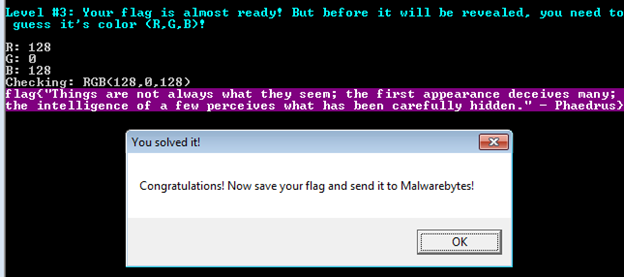To be honest, there was probably an easier way to beat that final challenge, but I am not too hot with cryptography. We knocked our bruteforce time down considerably and I feel that is pretty good too. Overall, this was a fun challenge that kept me guessing. Cannot wait for the next one!

As always, I am still fairly new to this, so if I did something wrong or if there was some other way I could have done this then please let me know! You can contact me on my Twitter or on LinkedIn.

Thanks for reading and happy reversing!

Reverse Engineering, Radare2, Hacking, MalwareBytes, CrackMe